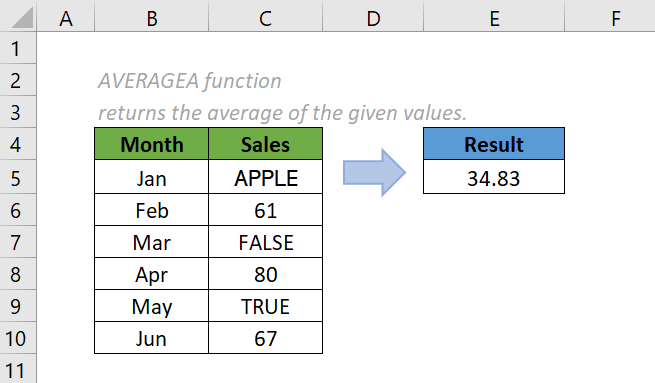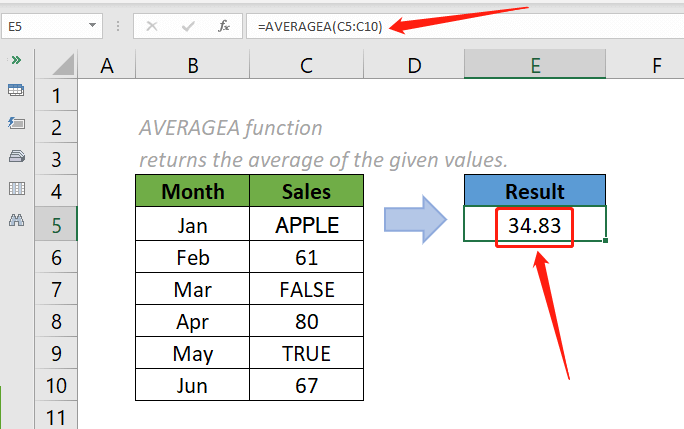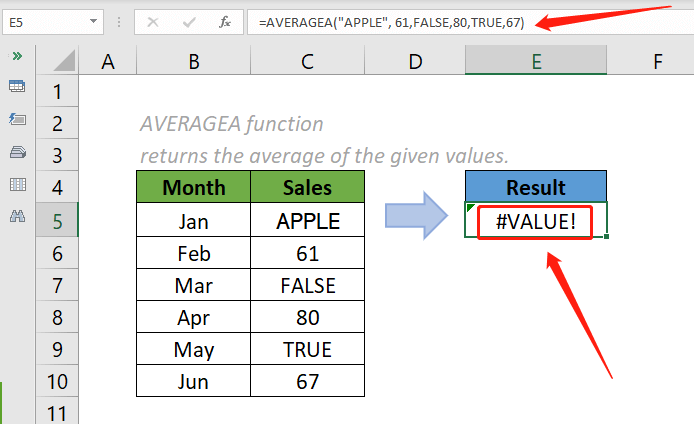## Excel AVERAGEA Function

The AVERAGEA function calculates the average (arithmetic mean) of the supplied values.

Unlike the AVERAGE function, it can evaluate cells that contain Logical values and text representations of numbers in the calculation, whereas the AVERAGE function just ignores these values.#### Syntax

=AVERAGEA (value1, [value2], ...)

#### Arguments

• Value1 (required): The first value, cell reference, or range.
• Value2, ... (optional): The subsequent values, cell references, or ranges.

#### Return value

The AVERAGEA function returns a numeric value.

#### Function notes

1. Arguments in the AVERAGEA function can be supplied as numbers, ranges, or cell references that contain values. Up to 255 arguments are allowed.
2. AVERAGEA function can count the Logical values and text representations of numbers (numbers enclosed with quotation marks, says “10”) that you type directly into the list of arguments.
3. AVERAGEA function ignores empty cells.
4. Unlike the AVERAGE function, cells that contain text or logical values can be counted in the AVERAGEA function.
5. AVERAGEA function evaluates TRUE as 1 and FALSE as zero. Cells with text, including the empty text "", text representations of numbers, and other text, are evaluated as zeros.
6. AVERAGEA function includes the zero values in the calculation.
7. . If you prefer the zero values to be ignored, use the AVERAGEIF or AVERAGEIFS function.
8. To calculate the average, all values are summed and divided by the count of numeric values in the AVERAGEA function.
9. The #VALUE! error value occurs if any of the supplied arguments you typed directly in the function are text strings that cannot be interpreted as numbers by Excel.

#### Examples

To calculate the average of the values provided in the below table, please copy the formula below into cell E5 and press the Enter key to get the result.

=AVERAGEA (C5:C10)Notes:

1. The argument in the above formula is supplied as a range that contain multiple values.
2. We can also use cell references as arguments of the formula. The formula above can be changed to:

=AVERAGEA (C5, C6, C7, C8, C9, C10)

3. To directly input the above values in the arguments of the formula is not recommended. The #VALUE! Error will occur when text that cannot be interpreted as a number are typed directly into the list of arguments.#### Relative Functions:

• Excel DEVSQ Function
The DEVSQ function calculates the sum of squares of the deviations from the sample mean.

• Excel DSTDEV Function
The DSTDEV function returns the estimated value of the standard deviation of a population based on a sample.

• Excel DSTDEVP Function
The Excel DSTDEVP function returns the standard deviation of a population by using the numbers from the entire database that match the given criteria you specify.

### The Best Office Productivity Tools

#### Kutools for Excel - Helps You To Stand Out From Crowd

 Popular Features: Find, Highlight or Identify Duplicates  |  Delete Blank Rows  |  Combine Columns or Cells without Losing Data  |  Round without Formula ... Super VLookup: Multiple Criteria  |  Multiple Value  |  Across Multi-Sheets  |  Fuzzy Lookup... Adv. Drop-down List: Easy Drop Down List  |  Dependent Drop Down List  |  Multi-select Drop Down List... Column Manager: Add a Specific Number of Columns  |  Move Columns  |  Toggle Visibility Status of Hidden Columns  |  Compare Columns to Select Same & Different Cells ... Featured Features: Grid Focus  |  Design View  |  Big Formula Bar  |  Workbook & Sheet Manager | Resource Library (Auto Text)  |  Date Picker  |  Combine Worksheets  |  Encrypt/Decrypt Cells  |  Send Emails by List  |  Super Filter  |  Special Filter (filter bold/italic/strikethrough...) ... Top 15 Toolsets:  12 Text Tools (Add Text, Remove Characters ...)  |  50+ Chart Types (Gantt Chart ...)  |  40+ Practical Formulas (Calculate age based on birthday ...)  |  19 Insertion Tools (Insert QR Code, Insert Picture from Path ...)  |  12 Conversion Tools (Numbers to Words, Currency Conversion ...)  |  7 Merge & Split Tools (Advanced Combine Rows, Split Excel Cells ...)  |  ... and more

Kutools for Excel Boasts Over 300 Features, Ensuring That What You Need is Just A Click Away...#### Office Tab - Enable Tabbed Reading and Editing in Microsoft Office (include Excel)

• One second to switch between dozens of open documents!
• Reduce hundreds of mouse clicks for you every day, say goodbye to mouse hand.
• Increases your productivity by 50% when viewing and editing multiple documents.
• Brings Efficient Tabs to Office (include Excel), Just Like Chrome, Edge and Firefox.